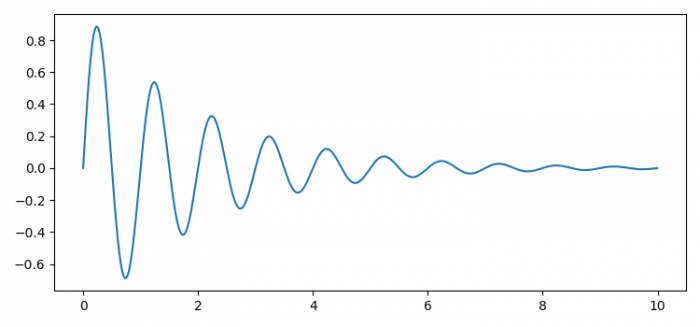# How can I get the length of a single unit on an axis in Matplotlib?

To get the length of a single unit on an axis in Matplotlib, we can take the following steps −

• Set the figure size and adjust the padding between and around the subplots.
• Create x and y data points using numpy.
• Create a new figure or activate an existing figure using figure() method.
• Add an '~.axes.Axes' to the figure as part of a subplot arrangement.
• Plot x and y data points using plot() method.
• To get the single unit length, use transData transform.
• Print the horizontal and vertical lengths.
• To display the figure, use show() method.

## Example

import numpy as np
import matplotlib.pyplot as plt

plt.rcParams["figure.figsize"] = [7.50, 3.50]
plt.rcParams["figure.autolayout"] = True

x = np.arange(0, 10, 0.005)
y = np.exp(-x / 2.) * np.sin(2 * np.pi * x)

fig = plt.figure()

ax.plot(x, y)

xy = ax.transData.transform([(0, 1), (1, 0)])\
-ax.transData.transform((0, 0))

print("Vertical length:", xy)
print("Horizontal length: ", xy)

plt.show()

## OutputIn addition to the plot, we will get the following output on the console −

Vertical length: 269.5
Horizontal length: 581.25